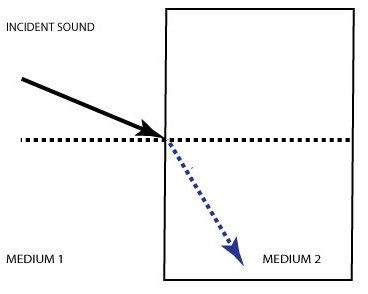##### Ultrasound Interactions

As a sound wave strikes an interface between two media with normal incidence (i.e orthogonal or perpendicular to the direction of the wave) sound can only either be reflected or transmitted.Remember that the due to conservation of energy, the intensity of the incident wave must equal the intensity of reflected sound + intensity of transmitted sound. Also note that the angle of incidence always equals the angle of reflection.

The proportion of sound which is reflected versus transmitted at the interface is determined by the relative impedance of the two media. Impedance is defined as the density of the media x speed of sound in the media. The greater the difference between the impedance of the 2 materials, the greater the proportion of sound which is reflected. This relationship is described by Snell’s law:

Sin(θ1) =     v1
Sin(θ2)        v2

This says that the ratio of the velocities of sound in the two media is equal to the ratio of the sines of the angle of incidence and refraction.

To use an extreme example, imagine an interface between air and a column of nitrogen gas. The impedance of the 2 gases are almost identical so almost all sound will be transmitted. In contrast, at the interface between air and brick, the impedances of brick and air are vastly different such that most sound is reflected.

When there is sound strikes an interface at oblique incidence rather than normal incidence, a third phenomenon can occur in addition to reflection and transmission: refraction. Refraction essentially describes bending of the sound wave as it strikes the interface. Refraction requires: 1) oblique incidence 2) A difference in the speed of sound in the two media.If speed in medium 2 is faster than medium 1, the angle of transmission will be greater than the angle of incidence.Likewise, if speed in medium 2 is slower than medium 1, the angle of transmission will be smaller than the angle of incidence